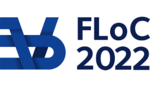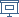FLOC 2022: FEDERATED LOGIC CONFERENCE 2022
FSCD ON WEDNESDAY, AUGUST 3RD
Days:
previous day
next day
all days

View: session overviewtalk overviewside by side with other conferences

08:30-09:00Coffee & Refreshments
09:00-10:30 Session 56B: Type Theory and Formalization
09:00
An Analysis of Tennenbaum's Theorem in Constructive Type TheoryPRESENTER: Marc Hermes

ABSTRACT. Tennenbaum's theorem states that the only countable model of Peano arithmetic (PA) with computable arithmetical operations is the standard model of natural numbers. In this paper, we use constructive type theory as a framework to revisit and generalize this result.

The chosen framework allows for a synthetic approach to computability theory, by exploiting the fact that, externally, all functions definable in constructive type theory can be shown computable. We internalize this fact by assuming a version of Church's thesis expressing that any function on natural numbers is representable by a formula in PA. This assumption allows for a conveniently abstract setup to carry out rigorous computability arguments and feasible mechanization.

Concretely, we constructivize several classical proofs and present one inherently constructive rendering of Tennenbaum's theorem, all following arguments from the literature. Concerning the classical proofs in particular, the constructive setting allows us to highlight differences in their assumptions and conclusions which are not visible classically. All versions are accompanied by a unified mechanization in the Coq proof assistant.

09:30
Constructing Unprejudiced Extensional Type Theories with Choices via ModalitiesPRESENTER: Vincent Rahli

ABSTRACT. Time-progressing expressions, i.e., expressions that compute to different values over time such as Brouwerian choice sequences or reference cells, are a common feature in many frameworks. For type theories to support such elements, they usually employ sheaf models. In this paper, we provide a general framework in the form of an extensional type theory incorporating various time-progressing elements along with a general possible-worlds forcing interpretation parameterized by modalities. The modalities can, in turn, be instantiated with topological spaces of bars, leading to a general sheaf model. This parameterized construction allows us to capture a distinction between theories that are “agnostic”, i.e., compatible with classical reasoning in the sense that classical axioms can be validated, and those that are “intuitionistic”, i.e., incompatible with classical reasoning in the sense that classical axioms can be proven false. This distinction is made via properties of the modalities selected to model the theory and consequently via the space of bars instantiating the modalities. We further identify a class of time-progressing elements that allows deriving “intuitionistic” theories that include not only choice sequences but also simpler operators, namely reference cells.

10:00
Division by two, in homotopy type theoryPRESENTER: Samuel Mimram

ABSTRACT. Natural numbers are isomorphism classes of finite sets and one can look for operations on sets which, after quotienting, allow recovering traditional arithmetic operations. Moreover, from a constructivist perspective, it is interesting to study whether those operations can be performed without resorting to the axiom of choice (the use of classical logic is usually necessary). Following the work of Bernstein, Sierpiński, Doyle and Conway, we study here ``division by two'' (or, rather, regularity of multiplication by two). We provide here a full formalization of this operation on sets, using the cubical variant of Agda, which is an implementation of the homotopy type theory setting, thus revealing some interesting points in the proof. Moreover, we show that this construction extends to general types, as opposed to sets.

10:30-11:00Coffee Break
11:00-12:30 Session 58C: Applications: Concurrency, Quantum Computation
11:00
PRESENTER: Siva Somayyajula

ABSTRACT. In sequential functional languages, sized types enable termination checking of programs with complex patterns of recursion in the presence of mixed inductive-coinductive types. In this paper, we adapt sized types and their metatheory to the concurrent setting. We extend the semi-axiomatic sequent calculus, a subsuming paradigm for futures-based functional concurrency, and its underlying operational semantics with recursion and arithmetic refinements. The latter enables a new and highly general sized type scheme we call sized type refinements. As a widely applicable technical device, we type recursive programs with infinitely deep typing derivations that unfold all recursive calls. Then, we observe that certain such derivations can be made infinitely wide but finitely deep. The resulting trees serve as the induction target of our strong normalization result, which we develop via a novel logical relations argument.

11:30
Addition and differentiation of ZX-diagramsABSTRACT. The ZX-calculus is a powerful framework for reasoning in quantum computing. It provides in particular a compact representation of matrices of interests. A peculiar property of the ZX-calculus is the absence of a formal sum allowing the linear combinations of arbitrary ZX-diagrams. The universality of the formalism guarantees however that for any two ZX-diagrams, the sum of their interpretations can be represented by a ZX-diagram. We introduce the first general, inductive definition of the summation of ZX-diagrams, relying on the construction of controlled diagrams. Based on this summation techniques, we provide an inductive differentiation of ZX-diagrams.

Indeed, given a ZX-diagram with variables in the description of its angles, one can differentiate the diagram according to one of these variables. Differentiation is ubiquitous in quantum mechanics and quantum computing (e.g. for solving optimisation problems). Technically, differentiation of ZX-diagrams is strongly related to summation as witnessed by the product rules.

We also introduce an alternative, non inductive, differentiation technique rather based on the isolation of the variables. Finally, we apply our results to deduce a diagram for an Ising Hamiltonian.

12:30-14:00Lunch Break

Lunch will be held in Taub lobby (CP, LICS, ICLP) and in The Grand Water Research Institute (KR, FSCD, SAT).

14:00-15:30 Session 59B: Automata and Computability
14:00
Restricting tree grammars with term rewriting
PRESENTER: Felix Laarmann

ABSTRACT. We investigate the problem of enumerating all terms generated by a tree-grammar which are also in normal form with respect to a set of directed equations (rewriting relation). To this end we show that deciding emptiness and finiteness of the resulting set is EXPTIME-complete. The emptiness result is inspired by a prior result by Common and Jacquemard on ground reducibility. The finiteness result is based on modification of pumping arguments used by Common and Jacquemard. We highlight practical applications and limitations. We provide and evaluate a prototype implementation. Limitations are somewhat surprising in that, while deciding emptiness and finiteness is EXPTIME-complete for linear and nonlinear rewrite relations, the linear case is practically feasible while the nonlinear case is infeasible, even for a trivially small example. The algorithms provided for the linear case also improve on prior practical results by Kallat et al.

14:30
On Lookaheads in Regular Expressions with BackreferencesPRESENTER: Nariyoshi Chida

15:00
Certified Decision Procedures for Two-Counter MachinesABSTRACT. Two-counter machines, pioneered by Minsky in the 1960s, constitute a particularly simple, universal model of computation. Universality of reversible two-counter machines (having a right-unique step relation) has been shown by Morita in the 1990s. Therefore, the halting problem for reversible two-counter machines is undecidable. Surprisingly, this statement is specific to certain instruction sets of the underlying machine model.

In the present work we consider two-counter machines (CM2) with instructions inc(c) (increment counter c, go to next instruction), dec(c,q) (if counter c is zero, then go to next instruction, otherwise decrement counter c and go to instruction q). While the halting problem for CM2 is undecidable, we give a decision procedure for the halting problem for reversible CM2, contrasting Morita's result.

We supplement our result with decision procedures for uniform boundedness (is there a uniform bound on the number of reachable configurations?) and uniform mortality (is there a uniform bound on the number of steps in any run?) for CM2.

Termination and correctness of each presented decision procedure is certified using the Coq proof assistant. In fact, both the implementation and certification is carried out simultaneously using the tactic language of the Coq proof assistant. Building upon existing infrastructure, the mechanized decision procedures are contributed to the Coq library of undecidability proofs.

15:30-16:00Coffee Break
16:00-17:30 Session 61B: Lambda Calculus and Normalization/Reduction
16:00
Strategies for Asymptotic Normalization
PRESENTER: Giulio Guerrieri

ABSTRACT. We introduce a technique to study normalizing strategies when termination is asymptotic, that is, the result appears as a limit, as opposite to reaching a normal form in a finite number of steps. Asymptotic termination occurs in several settings, such as effectful, and in particular probabilistic computation---where the limits are distributions over the possible outputsor infinitary lambda-calculi---where the limits are infinitary normal forms such as Böhm trees.

As a concrete application, we obtain a result which is of independent interest: a normalization theorem for Call-by-Value (and---in a uniform way---for Call-by-Name) probabilistic lambda-calculus.

16:30
Solvability for Generalized ApplicationsPRESENTER: Loïc Peyrot

ABSTRACT. Solvability is a key notion in the theory of call-by-name λ-calculus, used in particular to identify meaningful terms. However, adapting this notion to other call-by-name calculi, or extending it to different models of computation —such as call-by-value—, is not straightforward. In this paper, we study solvability for call-by-name and call-by-value λ-calculi with generalized applications, both variants inspired from von Plato’s natural deduction with generalized elimination rules. We develop an operational as well as a logical theory of solvability for each of them. The operational characterization relies on a notion of solvable reduction for generalized applications, and the logical characterization is given in terms of typability in an appropriate non-idempotent intersection type system. Finally, we show that solvability in generalized applications and solvability in the λ-calculus are equivalent notions.

17:00
PRESENTER: Willem Heijltjes

ABSTRACT. We present normalization for intuitionistic combinatorial proofs (ICPs) and relate it to the simply-typed lambda-calculus. We prove confluence and strong normalization.

Combinatorial proofs, or "proofs without syntax", form a graphical semantics of proof in various logics that is canonical yet complexity-aware: they are a polynomial-sized representation of sequent proofs that factors out exactly the non-duplicating permutations. Our approach to normalization aligns with these characteristics: it is canonical (free of permutations) and generic (readily applied to other logics).

Our reduction mechanism is a canonical representation of reduction in sequent calculus with closed cuts (no abstraction is allowed below a cut), and relates to closed reduction in lambda-calculus and supercombinators. While we will use ICPs concretely, the notion of reduction is completely abstract, and can be specialized to give a reduction mechanism for any representation of typed normal forms.

18:30-20:30 Walking tour (at Haifa)

pickup at 18:00 from the Technion (Tour at Haifa, no food will be provided)